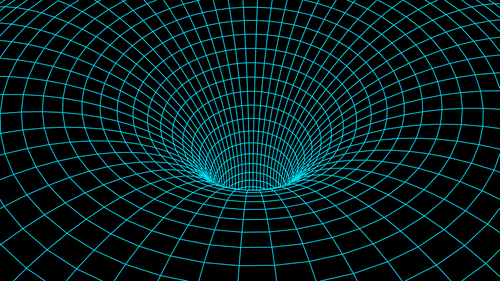Space and PhysicsPUBLISHED

# Quantum Computing And Holograms Might Tell Us What’s Inside A Black Hole6Comments340SharesWhat do you look like, black holes? Imager credit: Oleksii Lishchyshyn/Shutterstock.com

Black holes are objects at the limit of our understanding of physics. We can’t see what goes on inside them, beyond the event horizon, because their enormous gravity doesn't let anything, including light, escape. This means what we know if their inner properties is a matter of mathematical models, which can often fail to tell us what is really going on.

A new paper published in the journal PRX Quantum is attempting to build up the necessary mathematical scaffolding to do just that, bridging the current gap between quantum mechanics and general relativity, our leading theory of gravity. The two form the bedrock of our current understating of physics and yet don’t seem to work together, so physicists are looking for the fabled quantum theory of gravity and one crucial approach is through the principle of holography.

"In Einstein's General Relativity theory, there are no particles — there's just space-time. And in the Standard Model of particle physics, there's no gravity, there's just particles," Enrico Rinaldi, from the University of Michigan, said in a statement. "Connecting the two different theories is a longstanding issue in physics — something people have been trying to do since the last century."

Many will be familiar with holograms, projections of beings, and things made of light. The general holographic idea is that all of the information of our three-dimensional universe can be encoded on a two-dimensional surface. This principle underpins the concept of holographic duality, a conjecture that creates a mathematical equivalence between the theory of gravity and the theory of particles — what happens mathematically in the theory of gravity happens in the theory of particles, and vice versa.

So, the three-dimensional gravity of black holes is translated to a two-dimensional interaction, a flat disk, of particles above them. To find the mathematical connection, researchers are using quantum matrix models, a representation of particle theory.

If the holographic duality is correct, if we understand the way particles behave we should understand how gravity behaves, so by finding the right model we could finally have a way to connect gravity and quantum mechanics.

However, if finding the right model was easy, it would have been done already. And that’s where advanced computing comes in. The team is using deep learning, sophisticated statistical methods, and even the principles of quantum computing to find the ground state — the state with the least possible energy — among all the possible quantum matrix models.

"It's really important to understand what this ground state looks like, because then you can create things from it," Rinaldi said. "So for a material, knowing the ground state is like knowing, for example, if it's a conductor, or if it's a superconductor, or if it's really strong, or if it's weak. But finding this ground state among all the possible states is quite a difficult task. That's why we are using these numerical methods."

The approaches can provide the full information of the ground state and allow the construction of a full model of at least one black hole.

“Because these matrices are one possible representation for a special type of black hole, if we know how the matrices are arranged and what their properties are, we can know, for example, what a black hole looks like on the inside," Rinaldi explained. "What is on the event horizon for a black hole? Where does it come from? Answering these questions would be a step towards realizing a quantum theory of gravity.”Space and Physics
•